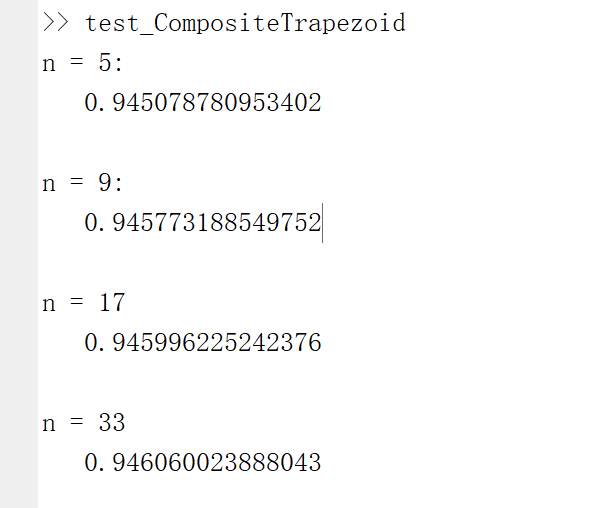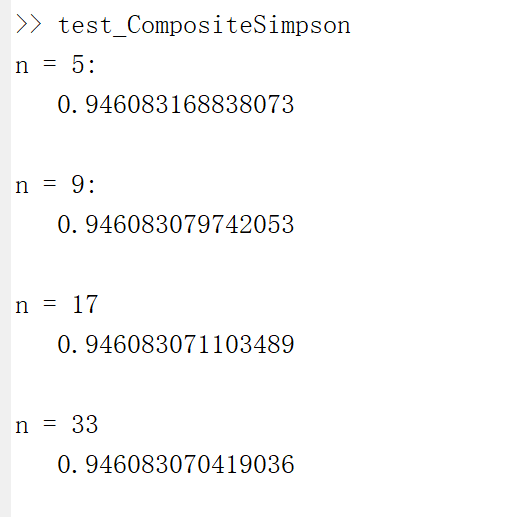# Halo

A magic place for coding

0%

## 介绍

这篇博客讨论的是一个数值积分问题，对于一些难于求积的函数，使用牛顿-莱布尼茨公式显然是不科学的。因此对于这类问题，我们可以从积分中值定理出发，使用矩形或梯形的面积去近似积分值。
这里主要讨论的是复合梯形公式复合辛普森公式，统称为复合求积法。这种通过把积分区间细分成若干个子区间（通常是等分），再在每个子区间上使用低阶求积公式，从而提高了计算精度。

## 分析

### 1.复合梯形公式

该公式核心思想是对于细分后的每一个子区间，使用梯形公式求积。假设将区间$[a,b]$等分为$n$个子区间，分点$x_k = a + kh，h = \frac{b-a}{n}，k=0,1,…,n$，公式的原理就是对于每一块小梯形而言，上底为$f(x_k)$，下底为$f(x_{k+1})$，高为$h$。
复合梯形公式如下：
$$I = \int^b_af(x)dx = \sum^{n-1}{k=0}\int^{x{k+1}}{x_k}f(x)dx = \frac{h}{2}\sum^{n-1}_{k=0}[f(x_k) + f(x_{k+1})]，$$

$$T_n = \frac{h}{2}\sum^{n-1}_{k=0}[f(x_k) + f(x_{k+1})] = \frac{h}{2}[f(a)+2\sum^{n-1} {k=1}f(x_k) + f(b)]$$

### 2.复合辛普森公式

复合辛普森公式的思路和复合梯形公式的思路一致，差别在于它的低阶求积公式使用了辛普森公式。辛普森公式是经过加权改造的梯形公式，集中在中间的点的权值更高，因此相比起简单的梯形公式，辛普森公式拥有更高的数值精度。
将区间&[a,b]&分为&n&等分，在每个字区间$[x_k, x_{k+1}]$上采用辛普森公式，记$x_{k+1/2} = x_k + \frac{1}{2}h$，得：
$$I = \int^b_af(x)dx = \sum^{n-1}{k=0}\int^{x{k+1}}{x_k}f(x)dx \ = \frac{h}{6}\sum^{n-1}_{k=0}[f(x_k) + 4f(x_{k+1/2}) + f(x{k+1})]$$

$$S_n = \frac{h}{6}[f(a) + 4\sum^{n-1}{k=0}f(x{k+1/2}) + 2\sum^{n-1}_{k=1}f(x_k) + f(b)]$$

## 代码实现

### 1.复合梯形公式

根据上述推到的复合梯形公式，编写matlab代码。

### 2.复合辛普森公式

根据上述推导的复合辛普森公式，编写matlab代码。

## 小结

采样点的数目分别为5，9，17，33，分别进行简单的数值实验，得出结果如下图：

1. 复合梯形公式2. 复合辛普森公式当然，除此之外还有著名的龙贝格求积公式高斯求积公式等，这篇博客只涉及到很小的一部分，以后有机会继续补充，谢谢！

#### 参考资料：

1.数值分析（第5版） 李庆扬，王能超，易大义

Welcome to my other publishing channels